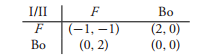### Solve this game and graph the rational reaction sets

Assignment Help Management Theories
##### Reference no: EM131445718

Question: Hawk-Dove Game. Two companies both want to take over a sales territory. They have the choices of defending the territory and fighting if necessary, or act as if willing to fight but if the opponent fights (F ), then backing off (Bo). They look at this as a two-person nonzero sum game with matrixSolve this game and graph the rational reaction sets.

#### Find the probability distribution of expected term

let A, B be 2 × 2 games and P2×2 = ( pij) a probability distribution. Show that P is a correlated equilibrium if and only if The payoffs in terms of expected social welfare ar

#### Define extensive form of given game

Convert the following extensive form game to a strategic form game. Be sure to list all of the pure strategies for each player and then use the matrix to find the Nash equil

#### Solve the game using gambit

a) Draw the extensive form of the game assuming the players choose simultaneously. (b) Use Gambit to solve the game and show that the mixed Nash Equilibrium is X∗ = (0.98159

#### Find the bimatrix

Suppose two merchants have to choose a location along the straight road. They may choose any point in {1, 2,..., n}. Assume there is exactly one customer at each of these po

#### What is latin square matrix

A Latin square game is a square game in which the matrix A is a Latin square. A Latin square of size n has every integer from 1 to n in each row and column. (a) Solve the g

#### Define submarine versus bomber game

A submarine (which occupies two squares) is trying to hide from a plane that can deliver torpedoes. The bomber can fire one torpedo at a square in the grid. If it is occupie

#### Formulate the game as a constant sum

Two farmers are having a fight over a disputed 6-yard-wide strip of land between their farms. Both farmers think that the strip is theirs. A lawsuit is filed and the judge o

#### Solve the game using linear program

In each of the following problems, set up the payoff matrices and solve the games using the linear programming method with both formulations, that is, both with and without

### Write a Review